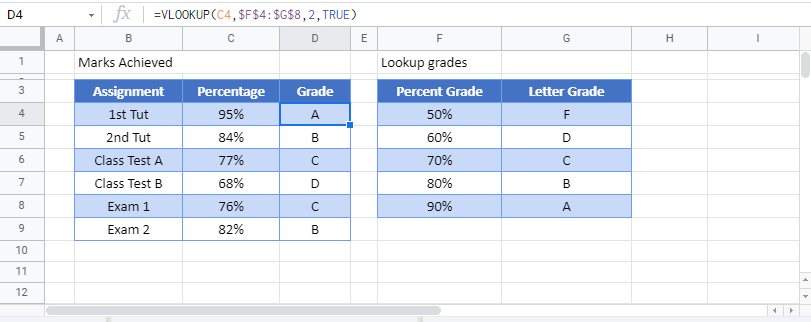This tutorial will demonstrate how to lookup letter grades in Excel and Google Sheets.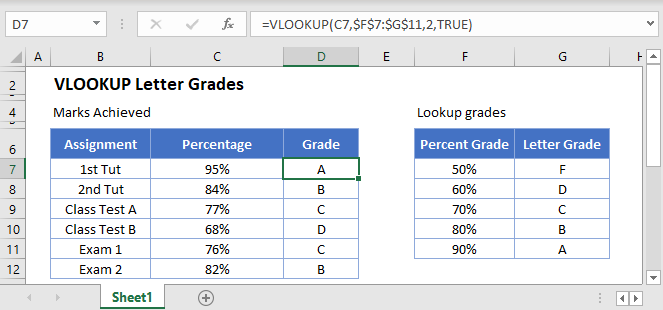To grade a score achieved in an assignment, we can use the VLOOKUP Function.

## VLOOKUP Function

The VLOOKUP Function searches for a value in the leftmost column of a table and then returns a value a specified number of columns to the right from the found value.

Firstly, we would need to have a table with the lookup values in it.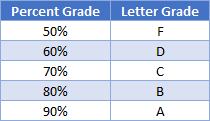The Percentage obtained will be in the first column, while the corresponding Letter Grade will be in the second column.  You will notice that the table is sorted from the lowest to the highest grade – this is important as you will see in the next section.

Next, we would type the VLOOKUP Formula where required.  In the syntax of the VLOOKUP, after we specify the column to return form the look up, we are then given the opportunity to look for an EXACT match, or an APPROXIMATE match.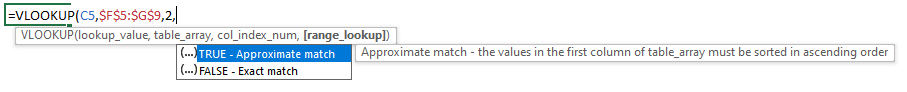Entering TRUE or FALSE as the last argument will either give us an EXACT match, or an APPROXIMATE match.

``=VLOOKUP(C5,\$F\$5:\$G\$9,2,TRUE)``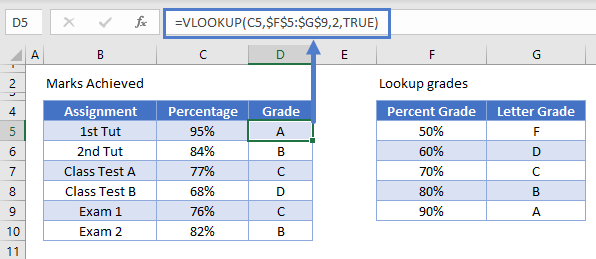The VLOOKUP will then look at the value in C5, and return the value in the 2nd column of the lookup range that closely matches the value in the 1st column of the lookup range – in this case the letter grade “A”.

When a VLOOKUP uses the TRUE argument for the match, it starts looking up from the BOTTOM of the table, which is the reason it is so important to have the table sorted from smallest to largest to obtain the correct letter grade.

As we have used an absolute reference (F4 or the \$ sign) for the table – \$F\$5:\$G\$9, we can copy this formula down from row 5 to row 10 to obtain the grades for each of the assignments.

<<For More Detail you can walk Through Our grade-formulas article.>>

HI Steven – Please review  above.

The example explained above works the same in Google sheets as it does in Excel.